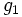# Geodesic mapping

(diff) ← Older revision | Latest revision (diff) | Newer revision → (diff)
Let$M$ and$N$ be Riemannian manifolds. A geodesic mapping from$M$ to$N$ is a diffeomorphism from$M$ to$N$ that sends geodesics to geodesics, such that its inverse also sends geodesics to geodesics.
Another way of saying this is that if$M$ is a differential manifold, then two Riemannian metrics$g_1$ and$g_2$ of$M$ are related by a geodesic mapping if the geodesics for$g_1$ are precisely the same as the geodesics for$g_2$.